# GSEB Solutions Class 10 Maths Chapter 14 Statistics Ex 14.3

Gujarat Board GSEB Solutions Class 10 Maths Chapter 14 Statistics Ex 14.3 Textbook Questions and Answers.

## Gujarat Board Textbook Solutions Class 10 Maths Chapter 14 Statistics Ex 14.3

Question 1.
The following frequency distribution gives the monthly consumption of electricity of 68 consumers of a locality. Find the median, mean and mode of the data and compare them.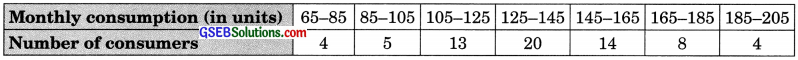Solution:
Median: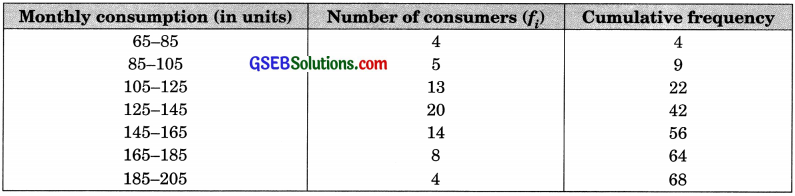n = 68
⇒ $$\frac {n}{2}$$ = 34
⇒ Median class 125 – 145.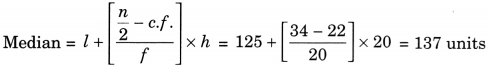Mean: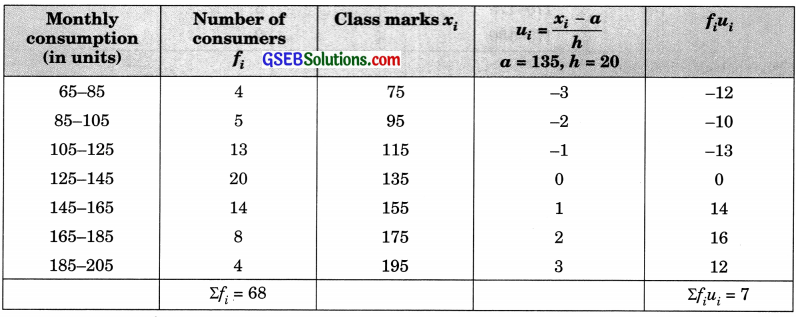Mean $$\bar{x}$$ = l + $$\frac{f_{i}-f_{0}}{2 f_{i}-f_{0}-f_{2}}$$ x h = 135 + $$\frac {7}{68}$$ x 20 = 135 + 2.06 = 137.06 units
Mode: Since the maximum frequency is 20.
Hence, the modal class is 125 – 145.
Mode = l + $$\left[\frac{f_{1}-f_{0}}{2 f_{1}-f_{0}-f_{2}}\right]$$ x h = 125 + $$\frac {7}{68}$$ x 20 = 125 + $$\frac{7}{40-27}$$ x 20
= 125 + $$\frac{7 x 20}{13}$$ = 125 + 10.77 = 135.77 unitsQuestion 2.
If the median of the distribution given below is 28.5, find the values of x and y. ∑fi = 60.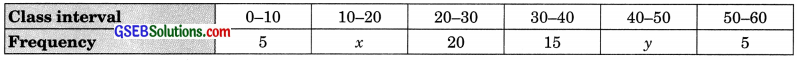Solution: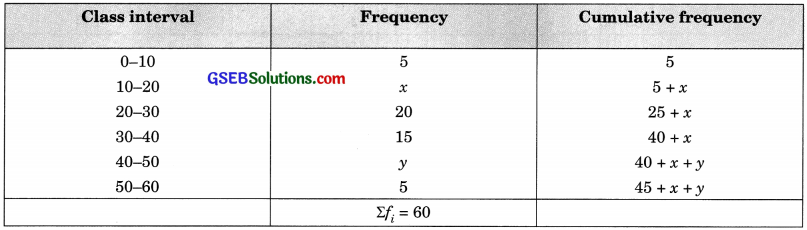Here, n = 60
⇒ x + y + 45 = 60
⇒  x + y = 15 ……(1)
Since median = 28.5
Hence, the median class is 20-30.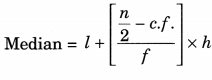28.5 = 20 + $$\left[\frac{30-(5+x)}{20}\right]$$ x 10
8.5 = $$\frac{25-x}{2}$$
⇒ 17 = 25 – x
⇒ x = 8
From (1) y = 15 – 8 = 7
∴ x = 8 and y = 7Question 3.
A life insurance agent found the following data for the distribution of ages of 100 policyholders. Calculate the median age, if polices are only given to persons having age 18 years onwards but less than 60 years.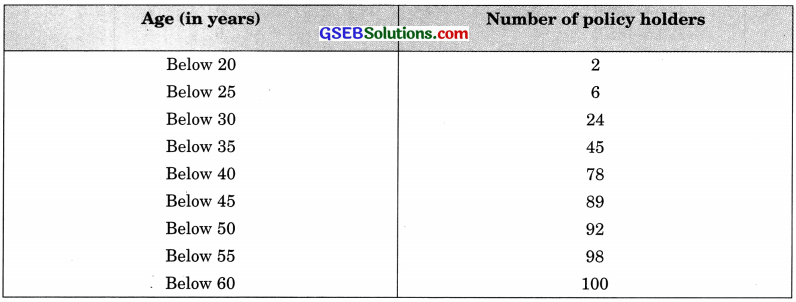Solution: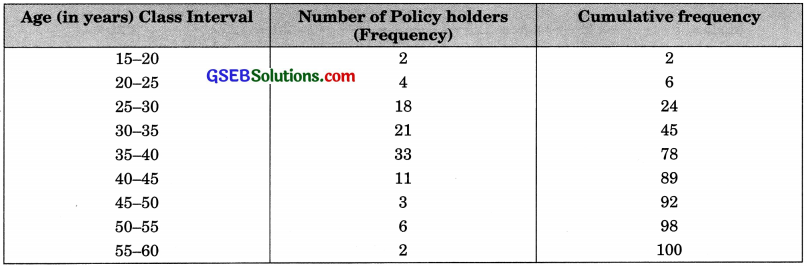n = 100
$$\frac{n}{2}$$ = $$\frac{100}{2}$$ = 50
Median class is 35 – 40.= 35 + $$\left[ \frac { 50-45 }{ 33 } \right]$$ x 5 = 35 + $$\frac{25}{33}$$ = 35 + 0.76 = 35. 76 yearsQuestion 4.
The lengths of 40 leaves of a plant are measured correct to the nearest millimeter, and the data obtained is represented in the following table: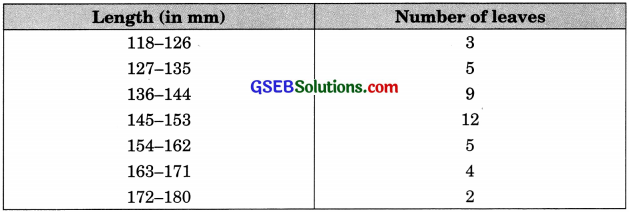Find the median length of the leaves.
Solution:
Firstly, data needs to be converted into continuous classes.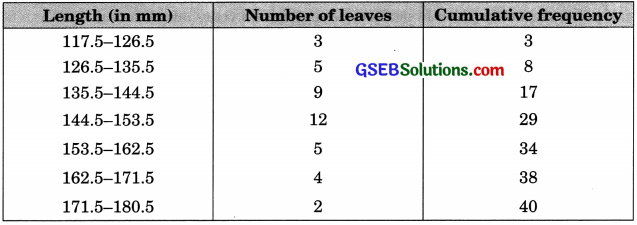Now, n = 40
So, $$\frac{n}{2}$$ = $$\frac{40}{2}$$ = 20
144.5 – 153.5 is the mediañ class.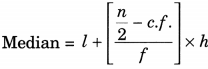= 144.5 + $$\left[ \frac { 20-17 }{ 12 } \right]$$ x 9 = 144.5 + 2.25 = 146.75 mm
Hence, the median length of the leaves is 146.75 mm.Question 5.
The following table gives the distribution of the lifetime of 400 neon lamps..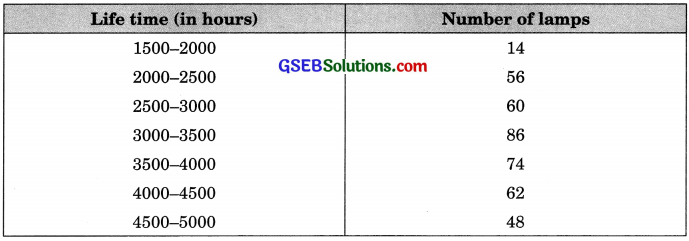Find the median lifetime of a lamp.
Solution: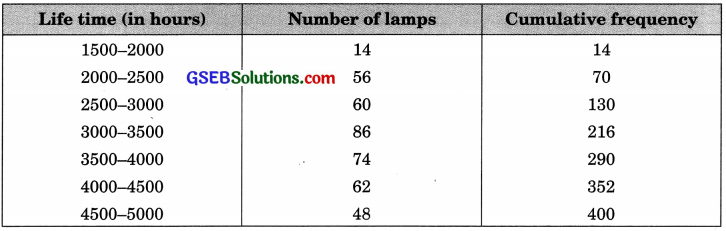Now, n = 400
So, $$\frac{n}{2}$$ = $$\frac{400}{2}$$ = 200= 3000 +1 1×500 = 3000 + 406.98 = 3406.98 hours
Hence, the median lifetime of a lamp is 3406.98 hours.Question 6.
100 surnames were randomly picked up from a local telephone directory and the frequency distribution of the number of letters in the English alphabets in the surnames was obtained as follows: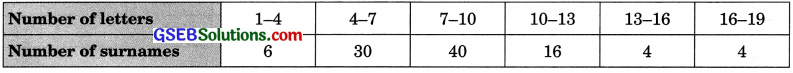Determine the median number of letters in the surnames. Find the mean number of letters in the surnames. Also, find the modal size of the surnames.
Solution:
Median: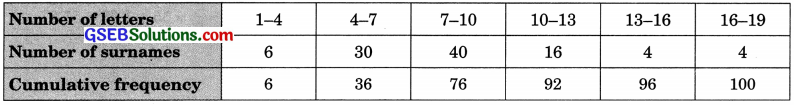Now, n = 100
So, $$\frac{n}{2}$$ = $$\frac{100}{2}$$ = 50
7 – 10 is the median class.= 7 + $\frac{50 – 36}{40}$ x 3 = 7 + $$\frac{21}{20}$$ = 7 + 1.05 = 8.05
Hence, the median number of letters in the surnames is 8.05.
Mean: Take a = 8.5, h = 3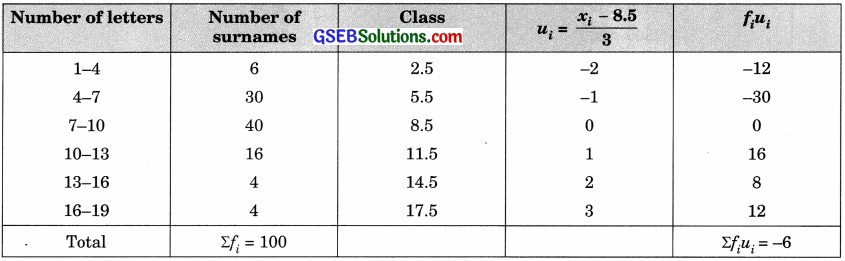$$\frac{21}{20}$$ = 8.5 + (-$$\frac{6}{100}$$) x 3 = 8.5 – 0.18 = 8.32
Hence, the mean number of letters in the surnames is 8.32.
Mode: Since the maximum number of surnames have a number of letters in the interval 7 – 10, the modal class is 7 – 10.
Therefore, l = 7, h = 3, f1 = 40, f0 = 30, f2 = 16
∴ Mode = l + $$\left(\frac{f_{1}-f_{0}}{2 f_{1}-f_{0}-f_{2}}\right)$$ x h = 7 + $$\left(\frac{40-30}{2 \times 40-30-16}\right)$$ x 3 = 7 + $$\frac{30}{34}$$ = 7 + 0.88 = 7.88
Hence, the modal size of the surnames is 7.88.Question 7.
The distribution below gives the weights of 30 students of a class, find the median weight of the students.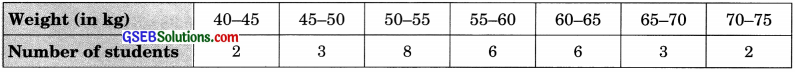Solution: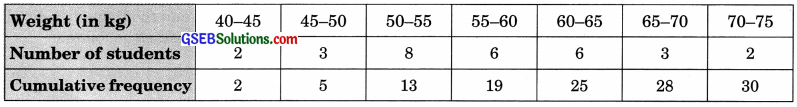Now, n = 30
So, $$\frac{n}{2}$$ = $$\frac{30}{2}$$ = 15= 55 + $$\frac{15 – 13}{6}$$ x 5 = 55 + $$\frac{10}{6}$$ = 55 + $$\frac{5}{3}$$ = 55 + 1.67 = 56.67
Hence, the median weight of the students is 56.67 kg.